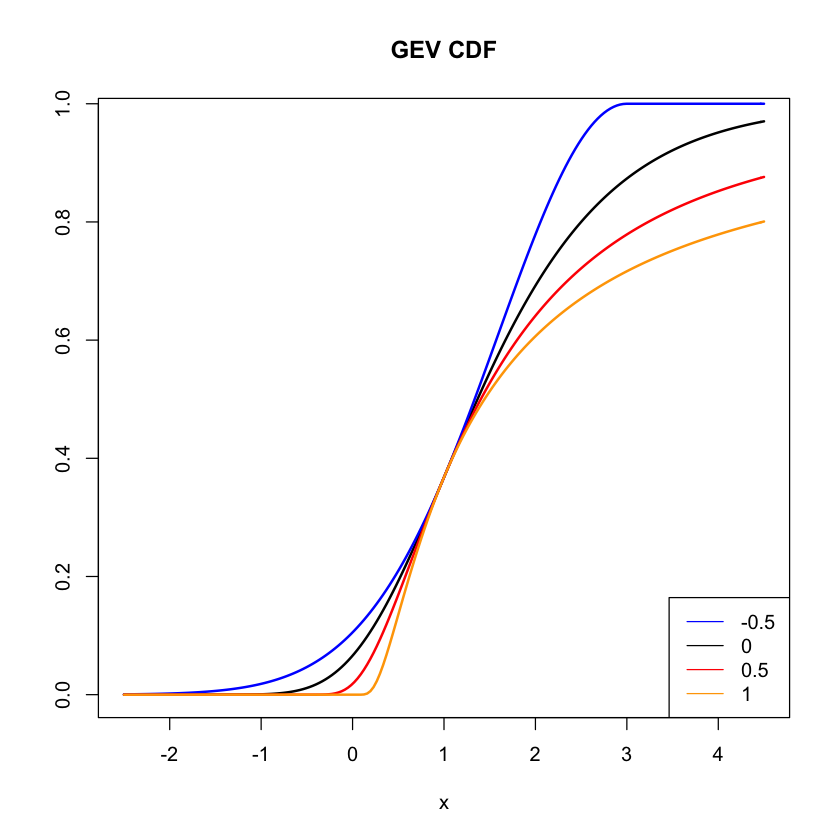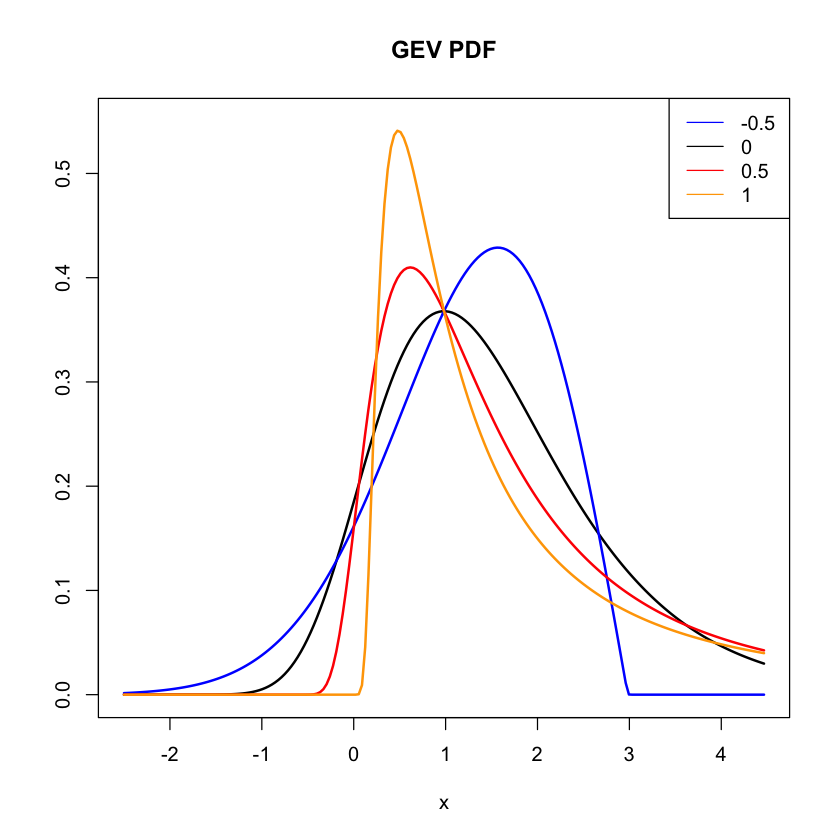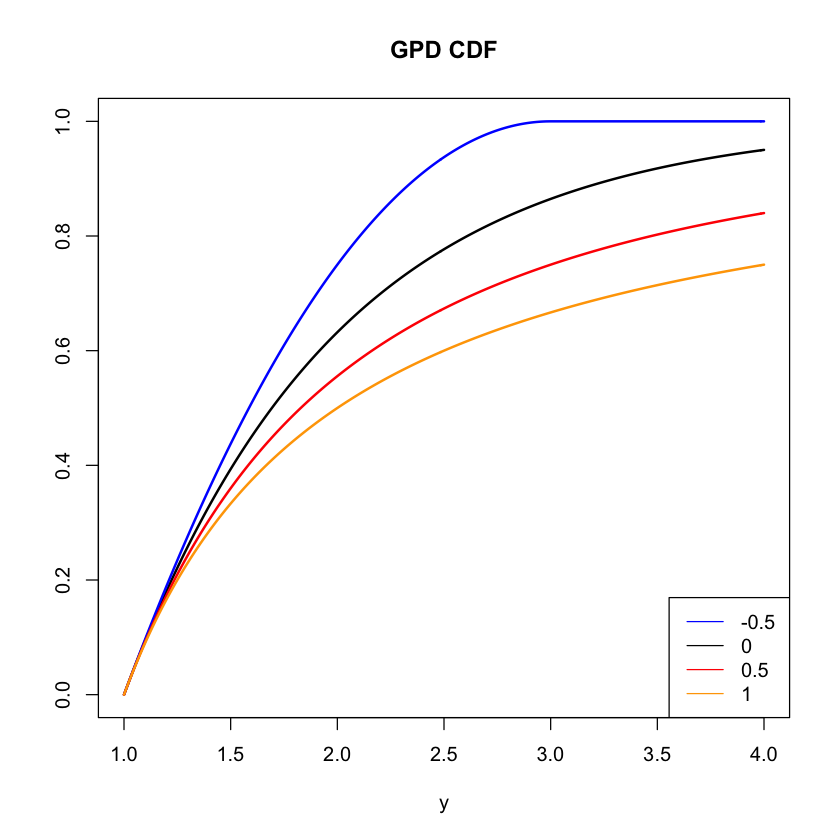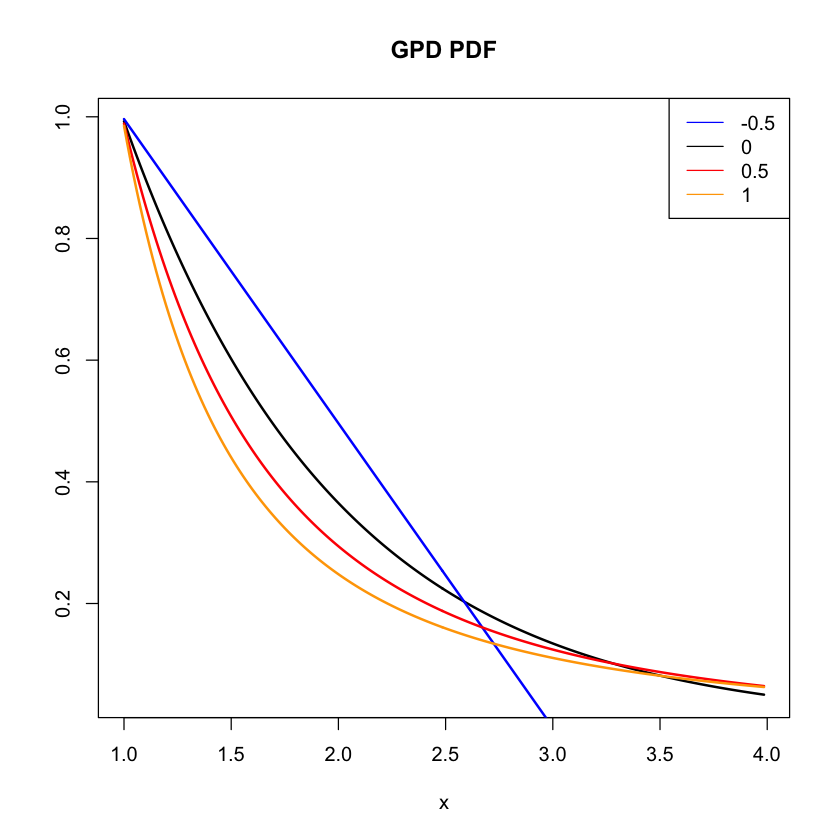# Extreme Value Distributions¶

Mar. 2, 2019 $\newcommand{\bs}{\boldsymbol}$ $\newcommand{\argmin}{\underset{\bs{#1}}{\text{arg min}}\,}$ $\newcommand{\argmax}{\underset{\bs{#1}}{\text{arg max}}\,}$ $\newcommand{\tr}{^{\top}}$ $\newcommand{\norm}{\left|\left|\,#1\,\right|\right|}$ $\newcommand{\given}{\,|\,}$ $\newcommand{\st}{\,\big|\,}$ $\newcommand{\E}{\mathbb{E}\left[#1\right]}$ $\newcommand{\P}{\mathbb{P}\left(#1\right)}$ $\newcommand{\abs}{\left|#1\right|}$ $\newcommand{\blue}{{\color{blue} {#1}}}$ $\newcommand{\red}{{\color{red} {#1}}}$ $\newcommand{\orange}{{\color{orange} {#1}}}$ $\newcommand{\pfrac}{\frac{\partial #1}{\partial #2}}$

Suppose we have i.i.d. sequence of random variables $X_1,X_2,...$ with a common distribution function $F(x)$. Let $M_n=\max(X_1,...,X_n)$ denote the maximum of first $n$ $X_i$'s. By independence, we have

$$\P{M_n\leq x} = F(x)^n \to 0 \quad \text{ as } n\to \infty.$$

To avoid this trivial case, we can normalize it by finding sequences $a_n$ and $b_n$ such that

$$\P{\frac{M_n-b_n}{a_n}\leq x} = F(a_nx+b_n)^n \to H(x),$$

where $H(x)$ is a non-degenerate distribution function. The Three Types Theorem proved by Gnedenko (1943) shows that if a non-degenerate $H$ exists, it must belong to the family of Generalized Extreme Value (GEV) distributions of the form

$$H(x) = \text{exp}\left\{-\left(1+\xi \frac{x-\mu}{\sigma}\right)_+^{-1/\xi} \right\}, \tag{1}$$

where $y_+= \max(y, 0)$, $\mu$ is the location parameter, $\sigma >0$ is the scale parameter, and $\xi$ is the shape parameter.

If $\xi\to 0$, we have the Gumbel distribution, which has the basic form

$$H(x) = \text{exp}\left\{-\text{exp}\left(-x \right) \right\}. \tag{2}$$

If $\xi >0$, we have the Frechet distribution, which has the basic form

$$H(x) = \begin{cases} 0 & x<0,\\ \text{exp}\left(-x^{-\alpha} \right) & x>0, \end{cases} \tag{3}$$

where $\alpha = 1/\xi$.

If $\xi <0$, we have the Weibull distribution, which has the basic form

$$H(x) = \begin{cases} \text{exp}\left(-|x|^\alpha \right), & x < 0, \\ 1, & x>0, \end{cases} \tag{4}$$

where $\alpha = -1/\xi$.

We can study how the shape parameter $\xi$ affects the distribution by fixing $\sigma=1$ and $\mu=1$.

In :
# Define GEV distribution function H(x)
GEV <- function(x, xi, mu, sig) {
if (xi==0){
exp(-exp(-(x-mu)/sig))
} else {
A <- 1 + xi*(x-mu)/sig
exp(-(max(A, 0))^(-1/xi))
}
}

In :
# Vectorize H(x) given a range of X values
GEV.vec <- function(xmin, xmax, xi, mu, sig) {
x <- seq(xmin, xmax, by=(xmax-xmin)/200)
sapply(1:length(x), function(i){GEV(x[i],xi,mu,sig)})
}

In :
# Plotting
xmin <- -2.5; xmax <- 4.5; mu <- 1; sig = 1
col <- c("blue", "red", "orange")
Xi <- c(-0.5, 0.5, 1)
x <- seq(xmin, xmax, by=(xmax-xmin)/200)
plot(x, GEV.vec(xmin, xmax, 0, mu, sig), type='l', ylab='', lwd=2, main="GEV CDF")

for (i in 1:length(Xi)) {
lines(x, GEV.vec(xmin, xmax, Xi[i], mu, sig), col=col[i], lwd=2)
}
legend("bottomright", legend=c("-0.5", "0", "0.5", "1"),
col=c("blue", "black", "red", "orange"), lty=1, cex=1)The figure above shows that a higher value of $\xi$ leads to a slower rise in the distribution function, implying a heavier tail. Instead of differentiating the expression (1), we can visualize the shape of the density functions numerically. Given arrays of values $(x_1,..., x_n)$ and $(f(x_1),..., f(x_n))$, the the shape of the derivative $f'(x)$ can be approximated by

$$f'(x_i) \approx \frac{f(x_{i+1})-f(x_i)}{x_{i+1}-x_i}, \quad i=1,...,n-1.$$

In :
y <- sapply(1:length(x), function(i){GEV(x[i],0,mu,sig)})
z <- sapply(1:(length(x)-1), function(i){(y[i+1]-y[i])/(x[i+1]-x[i])})
plot(x[1:200], z, type='l', xlab='x', ylab='', ylim=c(0, 0.55), lwd=2, main="GEV PDF")

for (j in 1:length(Xi)) {
y <- sapply(1:length(x), function(i){GEV(x[i],Xi[j],mu,sig)})
z <- sapply(1:(length(x)-1), function(i){(y[i+1]-y[i])/(x[i+1]-x[i])})
lines(x[1:200], z, col=col[j], lwd=2)
}
legend("topright", legend=c("-0.5", "0", "0.5", "1"),
col=c("blue", "black", "red", "orange"), lty=1, cex=1)Note that when $\xi=1, \mu=1, \sigma=1$, we have the unit Frechet distribution, whose support starts at $x=\mu-\sigma/\xi =0$. Also note that all PDFs intersect at $\mu=1$, as expected.

## Threshold Exceedance¶

Let $F(x)$ be the distribution function of $X$. We are interested in studying the probability distribution of $X$ conditioned on exceeding some threshhold $u$. Fix $u$ in the support of $X$, define

$$Y := X-u \given X>u.$$

The distribution function $G_u(y)$ of $Y$ can be expressed as

\begin{aligned} G_u(y)&=\P{X\leq u+y \given X>u} \\ &=\frac{\P{u<X \leq u+y}}{\P{X>u}} \\ &= \frac{F(u+y)-F(u)}{1-F(u)}. \end{aligned}

As $u\to x^+$, where $x^+$ is supremum of the support of $X$, and if $G_u \to G$ where $G$ is a non-degenerate distribution function, then $G$ must be the Generalized Pareto Distribution (GPD) of the form

$$G(y) = 1-\left(1+ \xi\left[\frac{y-\phi}{\psi}\right]_+ \right)_+^{-1/\xi}. \tag{5}$$

We see a lot of similarity between (1) and (5). In fact, it can be shown that a distribution function $F$ satisfies the assumptions for GEV if and only if it satisfies the assumption for GPD, and the shape parameter $\xi$ is the same in (1) and (5)!

If $\xi\to 0$, the GPD converges to the exponential distribution whose basic form is

$$G(y) = \begin{cases} 0 & y < 0 \\ 1-\text{exp}\left(-y\right) & y > 0 \end{cases}.\tag{6}$$

If $\xi>0$, we have the usual GPD of the basic form

$$G(y) = \begin{cases} 0 & y < 0 \\ 1-x^{-\alpha}, & y >0 \end{cases} \tag{7}$$

so the tail probability $1-G(x)$ decays like a power function $x^{-\alpha}$, where $\alpha=1/\xi$.

If $\xi <0$, the distribution will have a finite right boundary at $\phi-\psi/\xi$. The basic form (e.g. by setting $\phi=0, \psi=1, \xi=-1$) becomes

$$G(y) = \begin{cases} 0 & y <0 \\ 1-|y|^{\alpha} & 0 < y < 1 \\ 1 & y > 1. \end{cases} \tag{8}$$

We can repeat the same procedure to visualize this distribution.

In :
# Define GPD distribution function G(y)
GPD <- function(y, xi, phi, psi) {
if (xi==0){
max(1 - exp(-(y-phi)/psi), 0)
} else {
A <- (y-phi)/psi
B <- 1+xi*(max(A, 0))
1 - (max(B,0))^(-1/xi)
}
}

In :
# Vectorize G(y) given a range of Y values
GPD.vec <- function(xmin, xmax, xi, phi, psi) {
y <- seq(xmin, xmax, by=(xmax-xmin)/200)
sapply(1:length(y), function(i){GPD(y[i],xi,phi,psi)})
}

In :
# Plotting
ymin <- 1; ymax <- 4; phi <- 1; psi = 1
col <- c("blue", "red", "orange")
Xi <- c(-0.5, 0.5, 1)
y <- seq(ymin, ymax, by=(ymax-ymin)/200)
plot(y, GPD.vec(ymin, ymax, 0, phi, psi), type='l', ylab='', ylim=c(0,1), lwd=2, main="GPD CDF")

for (i in 1:length(Xi)) {
lines(y, GPD.vec(ymin, ymax, Xi[i], phi, psi), col=col[i], lwd=2)
}
legend("bottomright", legend=c("-0.5", "0", "0.5", "1"),
col=c("blue", "black", "red", "orange"), lty=1, cex=1)In :
yy <- sapply(1:length(y), function(i){GPD(y[i],0,phi,psi)})
zz <- sapply(1:(length(y)-1), function(i){(yy[i+1]-yy[i])/(y[i+1]-y[i])})
plot(y[1:200], zz, type='l', xlab='x', ylab='', lwd=2, main="GPD PDF")

for (j in 1:length(Xi)) {
yy <- sapply(1:length(y), function(i){GPD(y[i],Xi[j],phi,psi)})
zz <- sapply(1:(length(y)-1), function(i){(yy[i+1]-yy[i])/(y[i+1]-y[i])})
lines(y[1:200], zz, col=col[j], lwd=2)
}
legend("topright", legend=c("-0.5", "0", "0.5", "1"),
col=c("blue", "black", "red", "orange"), lty=1, cex=1)We see that the when $\xi$ is negative, the distribution has a finite right tail. When $\xi$ is positive, large value implies a heavier tail.

## Poisson-GPD Model¶

Recall that a homogeneous Poisson process is a stochastic process $N$ taking values in the nonnegative integers and

1. $N(0)=0$
2. If $0\leq s<t$, then $N(s)\leq N(t)$.
3. $N$ is a step process and all jumpts are of size 1.
4. $N(t)$ and $N(s+t)-N(s)$ are identically distributed.
5. $N(t_1), N(t_2-t_1)$ are independently distributed.
6. For each $t>0$, we have

\begin{aligned} P(N(t)=0) &= 1-\lambda t + O(t) \\ P(N(t)=1) &= \lambda t + O(t) \\ P(N(t)>1) &= O(t), \end{aligned}

where $O(t)$ is any function such that $O(t)/t \to 0$ as $t\to\infty$.

(As a side note, a stochastic process that satisfies properties 1-3 is called a counting process.) From these properties, it can be shown that for a given $t>0$, the random variable $N(t)$ follows a Poisson distribution with intensity $\lambda t$. We state it as a theorem below.

Theorem 1   Let $N(t)$ be a stochastic process satisfying properties 1-6. Then for each $t$,

$$N(t) \sim \text{Pois}(\lambda t).$$

Proof   For each $t$, $N(t)$ is a discrete random variable taking values in the nonnegative integers. Thus, its distribution is determined by its probability generating function

$$G(t, z) = \E{z^{N(t)}} = \sum_{n=0}^{\infty} z^n \P{N(t)=n}. \tag{9}$$

The probability generating function for a Poisson distribution is

$$G(t, z) = \sum_{n=0}^{\infty}z^n \frac{\lambda^n}{n!}e^{-\lambda} = e^{-\lambda(1-z)}. \tag{10}$$

It suffices to show that (9) converges to (10). We have that

\begin{aligned} & \quad G(t+\Delta t, z) - G(t, z) \\ &= \E{z^{N(t+\Delta t)}} - \E{z^{N(t)}} \\ &= \E{z^{N(t)}z^{N(t+\Delta t)-N(t)}} - \E{z^{N(t)}} \\ &= \E{z^{N(t)}}\E{z^{N(t+\Delta t)-N(t)}} - \E{z^{N(t)}} & [\text{Property } 5] \\ &= \E{z^{N(t)}}\E{z^{N(\Delta t)}} - \E{z^{N(t)}} & [\text{Property } 4] \\ &= \E{z^{N(t)}}\left(\E{z^{N(\Delta t)}} -1\right) \end{aligned} \tag{11}

Using property 6, we have,

\begin{aligned} & \quad \E{z^{N(\Delta t)}} \\ &= \P{N(\Delta t)=0} + z P(N(\Delta t) = 1) + \cdots \\ &= \left[1-\lambda\Delta t + O(\Delta t)\right] + \left[\lambda z \Delta t + O(\Delta t)\right] + O(\Delta t) \\ &= 1-\lambda(1-z)\Delta t + O(\Delta t). \end{aligned} \tag{12}

Combining (11) and (12), we have

\begin{aligned} & \quad G(t+\Delta t, z) - G(t, z) \\ &= -\left(\lambda(1-z)\Delta t - O(\Delta t)\right)G(t, z). \end{aligned} \tag{13}

Dividing (13) by $\Delta t$ and letting $\Delta t \to 0$, we have the following differential equation

$$\pfrac{G}{t}(t, z) = -\lambda(1-z)G(t, z).$$

The solution of this differential equation is

$$G(t, z) = Ce^{-\lambda t(1-z)},$$

where $C$ is a constant.

To solve for $C$, note that $G(0, z) = \E{z^{N(0)}} = 1 = C$. Hence we have that

$$G(t, z) = e^{-\lambda t(1-z)},$$

which is exactly the probability generating function for a Poisson distribution with intensity $\lambda t$. Great! $\blacksquare$

Now, suppose $X_1,X_2,..., X_n$ are i.i.d. random variables with distribution function $F(x)$. For a treshhold value $u$ in the support of $X_i$'s, we observe the indices $i$ for which $X_i > u$. In other words, for $i=1,..., n$, we define

$$U_i = \begin{cases} 1 & \text{ if } X_i > u \\ 0 & \text{ if } X_i < u. \end{cases}$$

If we rescale the indices of $U$ to $i/n$, then as $n\to \infty$, then binary sequence

$$U_{1/n}, U_{2/n},..., U_{n/n}$$

can be viewed as the occurrences of a counting process in the time interval $[0,1]$. Let $N(t) = \sum_{i<nt} U_{i/n}$. Then $N(t)$ follows a binomial distribution with parameters

$$N(t) \sim \text{Bin}\left(\lfloor nt \rfloor, 1-F(u)\right).$$

If we fix the expected value of this binomial random variable by choosing the appropriate threshold value $u$ and integer $n$, such that

$$\lambda = n(1-F(u)),$$

and letting $n\to\infty$, it is a well-known result that $N(t)$ converges to a Poisson distribution with intensity $\lambda t$.

Fix a unit time by setting $t=1$, we have the following joint Poisson-GPD process:

1. The number $N(1)$, which follows a Poisson distribution with intensity $\lambda$.
2. Given $N(1) \geq 1$, the values of the threshhold excess values $Y_1,..., Y_{N(1)}$ are i.i.d. random variables that follows a GPD distribution.

The Poisson-GPD model can provide a natural and beautiful connection between GPD and GEV distributions. For a value $x > u+\phi$ in the support of $X$, we have that

\begin{aligned} & \quad\, \P{\max_{i\leq N(1)} X_i \leq x } \\ &= \sum_{n=1}^{\infty} \P{N(1)=n, X_1 \leq x,...,X_n\leq x} \\ &= \sum_{n=1}^{\infty} \P{N(1)=n, Y_1 \leq x-u,...,Y_n\leq x-u} \\ &= \sum_{n=0}^{\infty} \frac{\lambda^n e^{-\lambda}}{n!}\cdot \left\{1-\left(1+\xi\left[\frac{x-u-\phi}{\psi}\right]_+\right)_+^{-1/\xi} \right\}^n \\ &= \text{exp}\left\{-\lambda\left(1+\xi\frac{x-u-\phi}{\psi}\right)_+^{-1/\xi}\right\}. \end{aligned}

Comparing that with distribution of GEV in (equation 1), we obtain the following link between these two sets of parameters:

\begin{aligned} \psi &= \sigma + \xi(u+\phi-\mu) \\ \lambda &=\left(1+\xi\frac{u+\phi-\mu}{\sigma}\right)^{-1/\xi}. \end{aligned} \tag{14}

For a simple illustration, suppose $X$ follows an exponential distribution with intensity $\lambda =1$. So $F(x)=1-e^{-x}$. We take sequences $a_n=1$ and $b_n=\log n$, then

\begin{aligned} F(a_nx+b_n)^n &= (1-e^{-x-\log n})^n \\ &= \left(1-\frac{e^{-x}}{n}\right)^n \\ &\to \text{exp}\left(-\text{exp}\left(-x)\right)\right), \end{aligned}

which is the Gumbel distribution defined in Equation (2). Hence, we have $\xi=0, \mu=0, \sigma=1$.

For treshhold exceedance,

\begin{aligned} G_u(y) &= \frac{F(u+y)-F(y)}{1-F(u)} \\ &= \frac{(1-e^{-u-y})-(1-e^{-u})}{1-(1-e^{-u})} \\ &= \frac{e^{-u}-e^{-u-z}}{e^{-u}} \\ &= 1-e^{-z}. \end{aligned}

This is also the exponential distribution. It makes sense since the exponential distribution is memoriless. From this we have $\phi=0$, and $\psi=1$. Finally from (14) we have that $\lambda =1$.